Homework Help Question & Answers

# Question 3 A researcher is interested in the relationship between the birth weights of infants and mothers' smoking habits. He uses the birth weight of an infant (ounces) and the average n...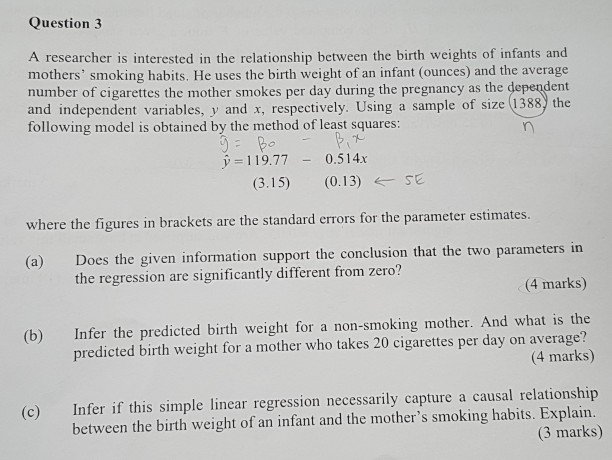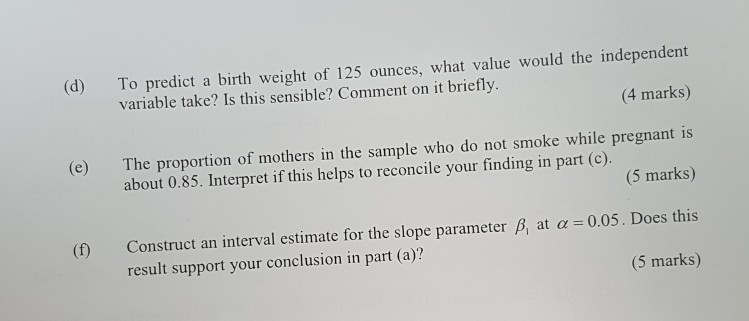Question 3 A researcher is interested in the relationship between the birth weights of infants and mothers' smoking habits. He uses the birth weight of an infant (ounces) and the average number of cigarettes the mother smokes per day during the pregnancy as the dependent and independent variables, y and x, respectively. Using a sample of size (1388 the following model is obtained by the method of least squares: y-119.770.514.x (3.15) (0.13) SE e the figures in brackets are the standard errors for the parameter estimates (a) Does the given information support the conclusion that the two parameters in the regression are significantly different from zero? (4 marks) Infer the predicted birth weight for a non-smoking mother. And what is the predicted birth weight for a mother who takes 20 cigarettes per day on average? (4 marks) (b) Infer if this simple linear regression necessarily capture a causal relationship between the birth weight of an infant and the mother's smoking habits. Explain (c) (3 marks)
(d) To predict a birth weight of 125 ounces, what value would the independent (4 marks) (e) The proportion of mothers in the sample who do not smoke while pregnant is (5 marks) ct an interval estimate for the slope parameter B, at a 0.05. Does this (5 marks) variable take? Is this sensible? Comment on it briefly. about 0.85. Interpret if this helps to reconcile your finding in part (c) result support your conclusion in part (a)?

#### Homework Answers

Answer #1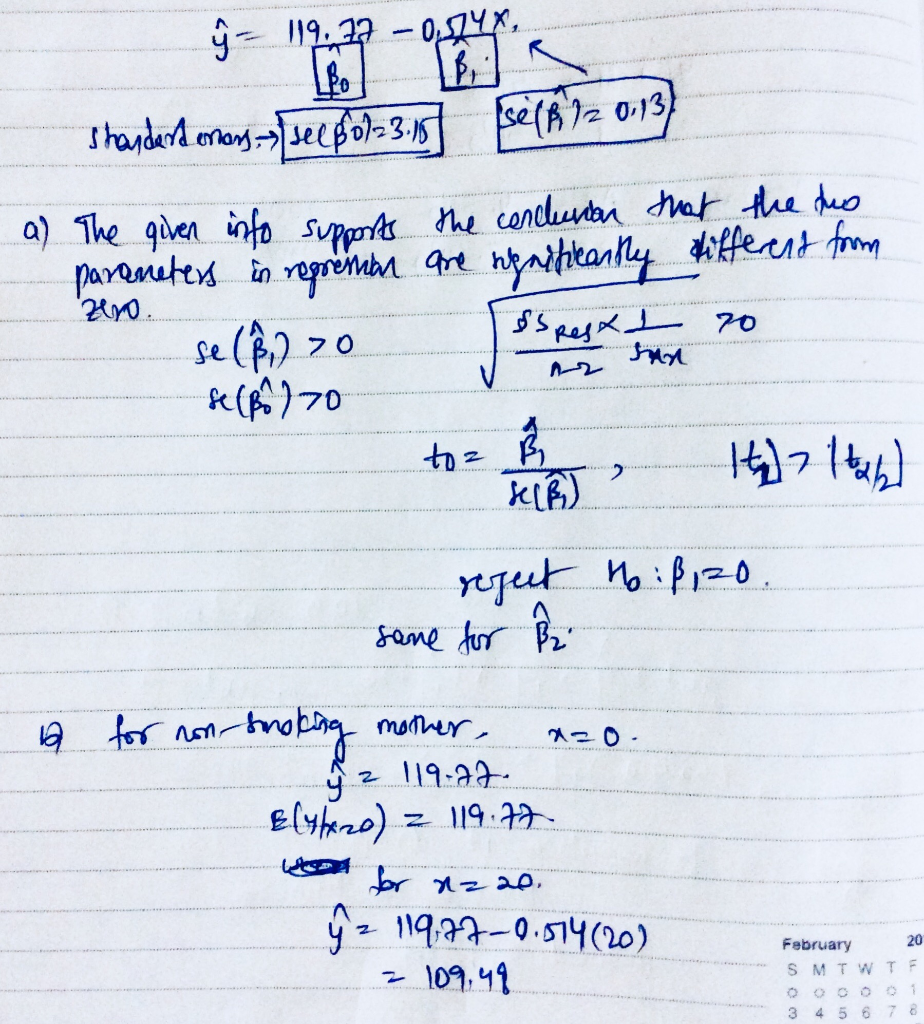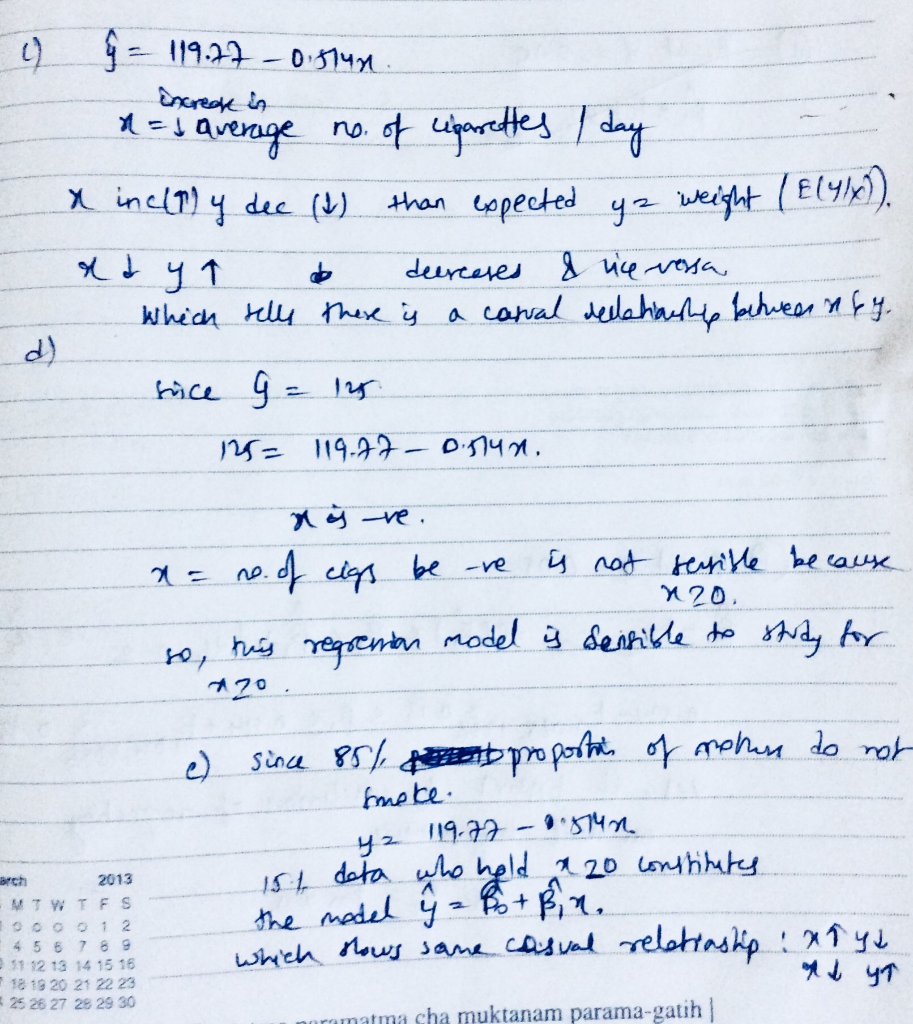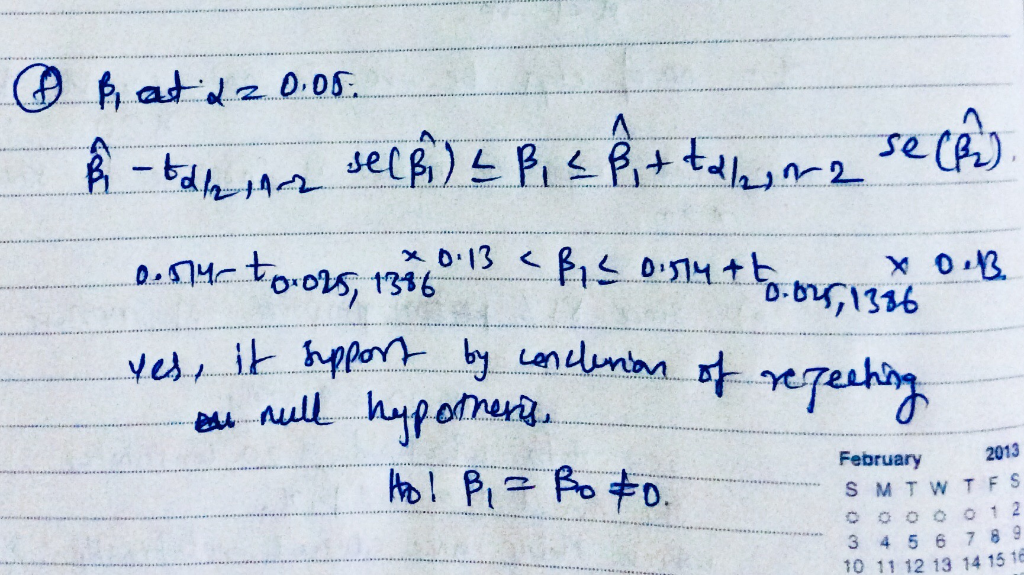Know the answer?
Your Answer:

#### Post as a guest

Your Name:

What's your source?

#### Earn Coin

Coins can be redeemed for fabulous gifts.

Not the answer you're looking for? Ask your own homework help question. Our experts will answer your question WITHIN MINUTES for Free.
Similar Homework Help Questions
• ### 1. Consider the following estimated outcome: bwght = 119.77-0.514cigs Where bwght-infant birth weight in ounces; cigs-average...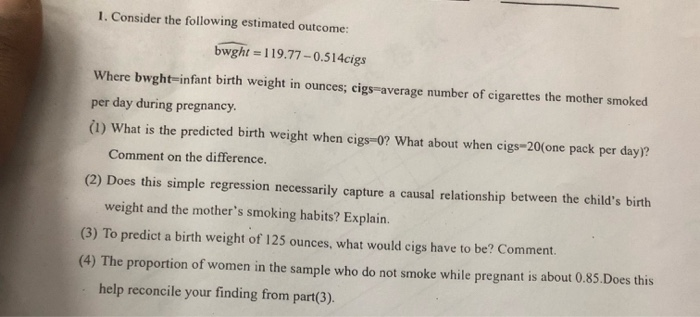1. Consider the following estimated outcome: bwght = 119.77-0.514cigs Where bwght-infant birth weight in ounces; cigs-average number of cigarettes the mother smoked per day during pregnancy. (1) What is the predicted birth weight when cigs=0? What about when cigs-20(one pack per day)? Comment on the difference. (2) Does this simple regression necessarily capture a causal relationship between the child's birth weight and the mother's smoking habits? Explain. (3) To predict a birth weight of 125 ounces, what would cigs have...

• ### A researcher is analysing the impact of smoking during pregnancy on infant health. Using a survey...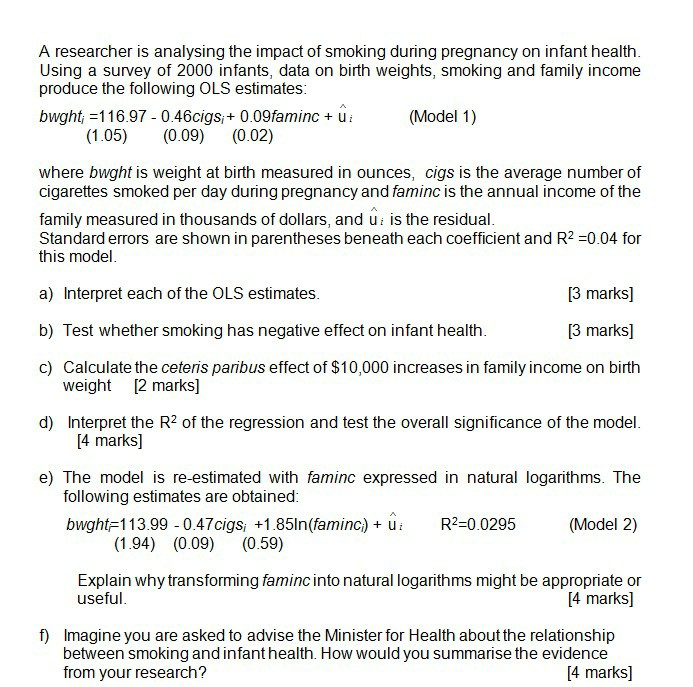A researcher is analysing the impact of smoking during pregnancy on infant health. Using a survey of 2000 infants, data on birth weights, smoking and family income produce the following OLS estimates: bwght =116.97 -0.46cigs; + 0.09faminc + lli (Model 1) (1.05) (0.09) (0.02) where bwght is weight at birth measured in ounces, cigs is the average number of cigarettes smoked per day during pregnancy and faminc is the annual income of the family measured in thousands of dollars, and...

• ### 3. (6 points) The dataset BWGT contains data on births to women in the United States....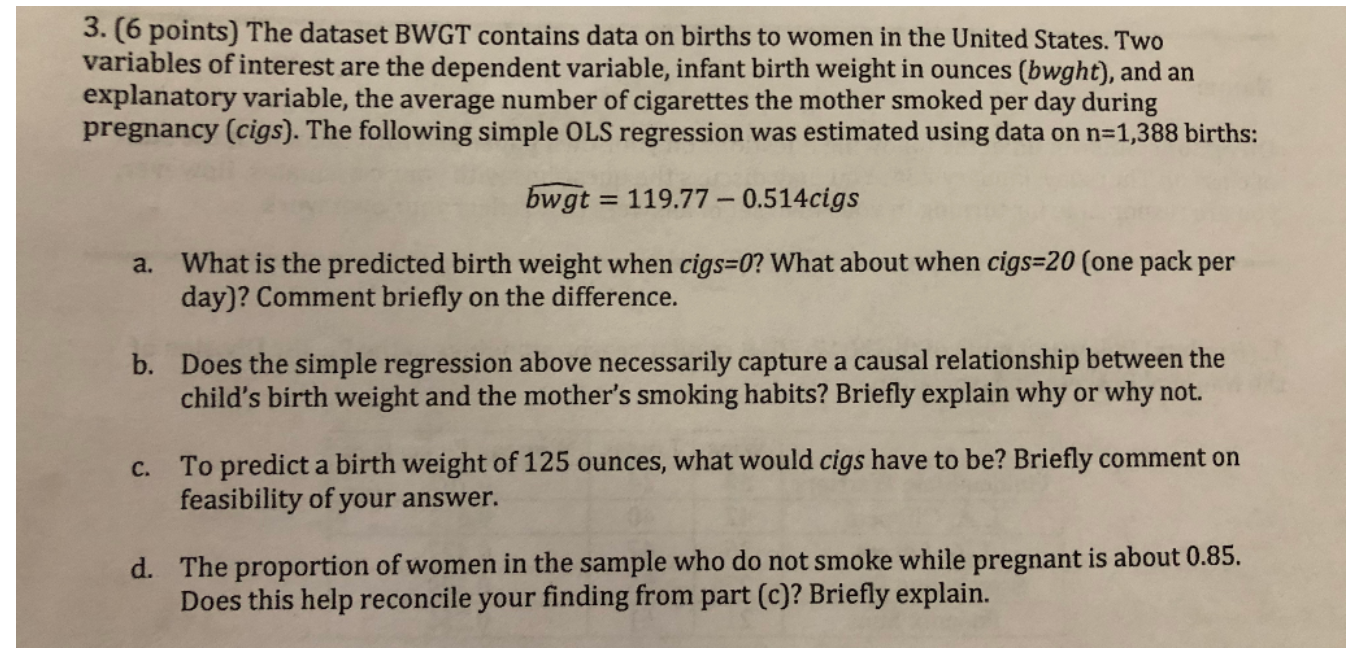3. (6 points) The dataset BWGT contains data on births to women in the United States. Two variables of interest are the dependent variable, infant birth weight in ounces (bwght), and an explanatory variable, the average number of cigarettes the mother smoked per day during pregnancy (cigs). The following simple OLS regression was estimated using data on n=1,388 births: bwgt = 119.77 – 0.514cigs a. What is the predicted birth weight when cigs=0? What about when cigs=20 (one pack per...

• ### 3. (6 points) The dataset BWGT contains data on births to women in the United States....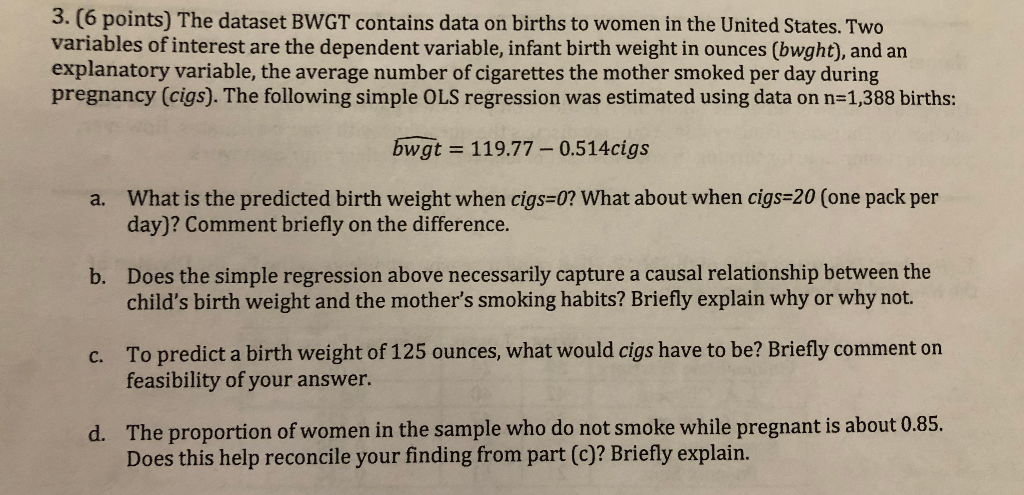3. (6 points) The dataset BWGT contains data on births to women in the United States. Two variables of interest are the dependent variable, infant birth weight in ounces (bwght), and an explanatory variable, the average number of cigarettes the mother smoked per day during pregnancy (cigs). The following simple OLS regression was estimated using data on n=1,388 births: bwgt = 119.77 – 0.514cigs What is the predicted birth weight when cigs=0? What about when cigs=20 (one pack per day)?...

• ### 4 The data set BWGHT contains data on births to women in the United States. Two...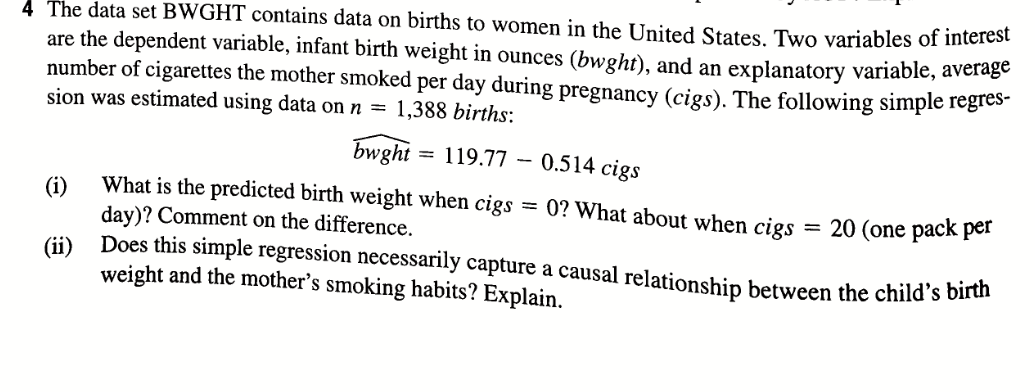4 The data set BWGHT contains data on births to women in the United States. Two variables of interest are the dependent variable, infant birth weight in ounces (bwght), and an explanatory variable, ava number of cigarettes the mother smoked per day during pregnancy (cigs). The following simple to sion was estimated using data on n = 1,388 births: bwght = 119.77 – 0.514 cigs (i) What is the predicted birth weight when cigs = 0? What about when cies...